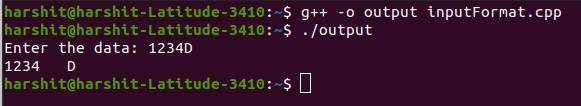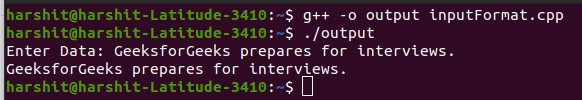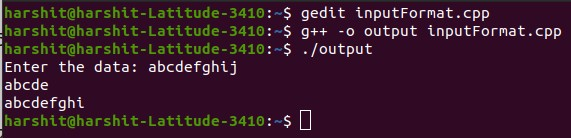Open In App

# Unformatted input/output operations In C++

In this article, we will discuss the unformatted Input/Output operations In C++. Using objects cin and cout for the input and the output of data of various types is possible because of overloading of operator >> and << to recognize all the basic C++ types. The operator >> is overloaded in the istream class and operator << is overloaded in the ostream class.

The general format for reading data from the keyboard:

cin >> var1 >> var2 >> …. >> var_n;

• Here, var1, var2, ……, varn are the variable names that are declared already.
• The input data must be separated by white space characters and the data type of user input must be similar to the data types of the variables which are declared in the program.
• The operator >> reads the data character by character and assigns it to the indicated location.
• Reading of variables terminates when white space occurs or character type occurs that does not match the destination type.

Program 1:

## C++

 `// C++ program to illustrate the``// input and output of the data``// entered by user``#include ``using` `namespace` `std;` `// Driver Code``int` `main()``{``    ``int` `data;``    ``char` `val;` `    ``// Input the data``    ``cin >> data;``    ``cin >> val;` `    ``// Print the data``    ``cout << data << ``"   "` `<< val;` `    ``return` `0;``}`

Output:Explanation: In the above program, 123 is stored in the variable val of integer, and B is passed to the next cin object and stored in the data variable of character.

### put() and get() functions:

The class istream and ostream have predefined functions get() and put(), to handle single character input and output operations. The function get() can be used in two ways, such as get(char*) and get(void) to fetch characters including blank spaces, newline characters, and tab. The function get(char*) assigns the value to a variable and get(void) to return the value of the character.

Syntax:

char data;

// get() return the character value and assign to data variable
data = cin.get();

// Display the value stored in data variable
cout.put(data);

Example:

char c;

// directly assign value to c
cin.get(c);

// Display the value stored in c variable
cout.put()

Program 2:

## C++

 `// C++ program to illustrate the``// input and output of data using``// get() and puts()``#include ``using` `namespace` `std;` `// Driver Code``int` `main()``{``    ``char` `data;``    ``int` `count = 0;` `    ``cout << ``"Enter Data: "``;` `    ``// Get the data``    ``cin.get(data);` `    ``while` `(data != ``'\n'``) {``        ``// Print the data``        ``cout.put(data);``        ``count++;` `        ``// Get the data again``        ``cin.get(data);``    ``}` `    ``return` `0;``}`

Output:### getline() and write() functions:

In C++, the function getline() and write() provide a more efficient way to handle line-oriented inputs and outputs. getline() function reads the complete line of text that ends with the new line character. This function can be invoked using the cin object.

Syntax:

cin.getline(variable_to_store_line, size);

The reading is terminated by the ‘\n’ (newline) character. The new character is read by the function, but it does not display it, instead, it is replaced with a NULL character. After reading a particular string the cin automatically adds the newline character at end of the string.

The write() function displays the entire line in one go and its syntax is similar to the getline() function only that here cout object is used to invoke it.

Syntax:

cout.write(variable_to_store_line, size);

The key point to remember is that the write() function does not stop displaying the string automatically when a NULL character occurs. If the size is greater than the length of the line then, the write() function displays beyond the bound of the line.

Program 3:

## C++

 `// C++ program to illustrate the``// input and output of file using``// getline() and write() function``#include ``#include ``using` `namespace` `std;` `// Driver Code``int` `main()``{``    ``char` `line;` `    ``// Get the input``    ``cin.getline(line, 10);` `    ``// Print the data``    ``cout.write(line, 5);``    ``cout << endl;` `    ``// Print the data``    ``cout.write(line, 20);` `    ``cout << endl;` `    ``return` `0;``}`

Output: